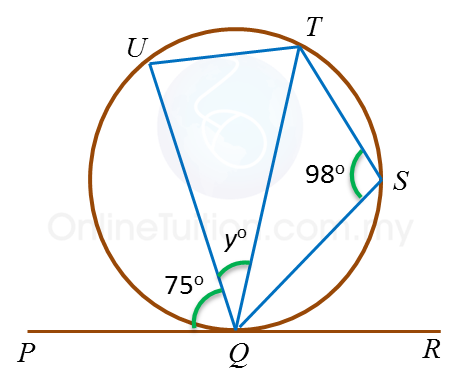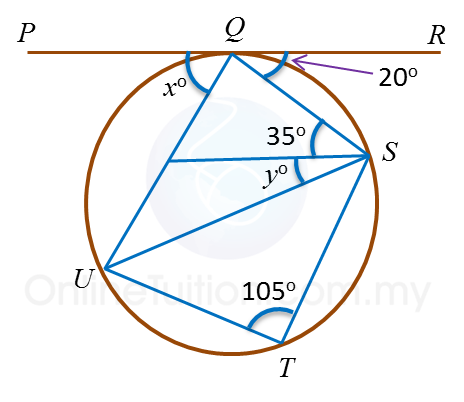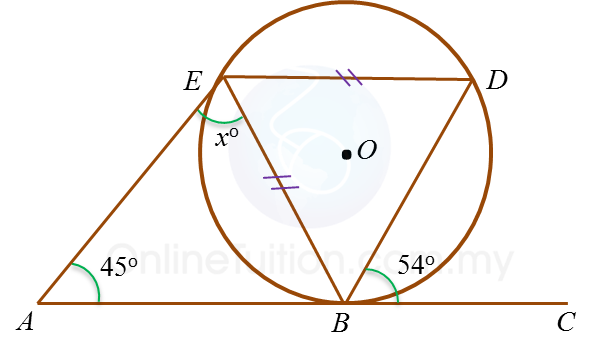# 6.4.2 Angles and Tangents of Circles (Sample Questions)

Example 1:
In the diagram, PQR is a tangent to the circle QSTU at Q.Find the value of y.

Solution:
Angle QUT
= 180o– 98o(opposite angle in cyclic quadrilateral QSTU )
= 82o
Angle QTU = 75o  ← (angle in alternate segment)
Therefore y= 180o – (82o + 75o)  ← (Sum of interior angles in ∆ QTU)
= 23o

Example 2:
In the diagram, PQR is a tangent to the circle QSTU at Q.Find the values of
(a) x   (b) y

Solution:
(a)
UTS + ∠UQS = 180o ←(opposite angle in cyclic quadrilateral QSTU)
105o + ∠ UQS = 180o
UQS = 75o
x+ 75o + 20o = 180o(the sum of angles on a straight line PQR = 180o)
x+ 95o = 180o
= 85o

(b)
PQU = ∠ QSU  ← (angle in alternate segment)
85o = 35o + y
y = 50o

Example 3:In the diagram, ABC is a tangent to the circle BDE with centre O, at B.
Find the value of x.

Solution:$\begin{array}{l}\angle BED=\angle CBD={54}^{\circ }\\ \angle BDE=\frac{{180}^{\circ }-{54}^{\circ }}{2}={63}^{\circ }←\overline{)\begin{array}{l}\text{Isosceles triangle}\\ \angle EBD=\angle EDB\end{array}}\\ \angle ABE=\angle BDE={63}^{\circ }\\ \text{In}△ABE,\\ {x}^{\circ }+{45}^{\circ }+{63}^{\circ }={180}^{\circ }\\ {x}^{\circ }+{108}^{\circ }={180}^{\circ }\\ x=72\end{array}$